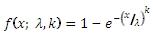# SQL Server WEIBULL.INV function

WEIBULLINV

Updated: 31 July 2010

Use WEIBULLINV to calculate the inverse of the cumulative distribution function of the Weibull distribution.

The formula for the cumulative distribution function is:Syntax
SELECT [wctStatistics].[wct].[WEIBULLINV] (
<@P, float,>
,<@Alpha, float,>
,<@Beta, float,>)
Arguments
@P
is the cumulative distribution function. @P is an expression of type float or of a type that implicitly converts to float
@Alpha
is the shape parameter of the distribution. @Alpha is an expression of type float or of a type that implicitly converts to float
@Beta
is the scale parameter of the distribution. @Beta is an expression of type float or of a type that implicitly converts to float
Return Types
float
Remarks
·         @P must be greater than zero and less than one (0 < @P < 1).
·         @Alpha must be greater than zero (0 < @Alpha).
·         @Beta must be greater than zero (0 < Beta).
Examples
Calculate the cumulative distribution function:
SELECT wct.WEIBULL(6, 1, 10, 'True')

This produces the following result

----------------------
0.451188363905974

(1 row(s) affected)

Calculate the inverse:
SELECT wct.WEIBULLINV(0.451188363905974,1, 10
This produces the following result
----------------------
6.00000000000001

(1 row(s) affected)

### SupportCopyright 2008-2023 Westclintech LLC         Privacy Policy        Terms of Service